This class provides thread-local variables. These variables differ from* their normal counterparts in that each thread that accesses one (via its* {@code get} or {@code set} method) has its own, independently initialized* copy of the variable. {@code ThreadLocal} instances are typically private* static fields in classes that wish to associate state with a thread (e.g.,* a user ID or Transaction ID).

## 看代码

public class Test {
private static int a = 10;
public static void main(String[] args) {

A.start();
B.start();

}

@Override
public void run() {
local.set(a+10);
local.remove();
}
}

@Override
public void run() {

}
}
}


private static int a = 10;


int a = 10;


 private static ThreadLocal<Integer> local;


static class ThreadA implements Runnable{
@Override
public void run() {
local.set(a+10);
local.remove();
}
}

@Override
public void run() {

}
}


local = new ThreadLocal();


ThreadLocal local = new ThreadLocal();


int a = 10;


local.set();



local.set(a+10);



System.out.println(local.get()+Thread.currentThread().getName());



local.get()



## 基本使用

ThreadLocal local = new ThreadLocal();
local.set(a+10);
local.get()



local.remove();



Thread A = new Thread(new ThreadA());
A.start();
B.start();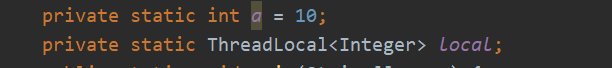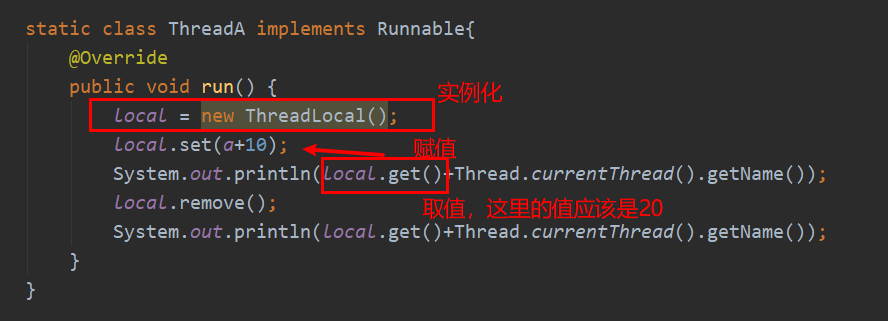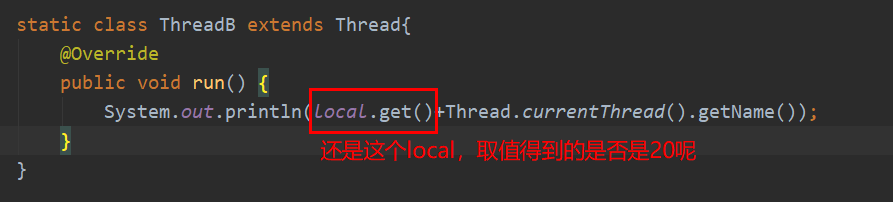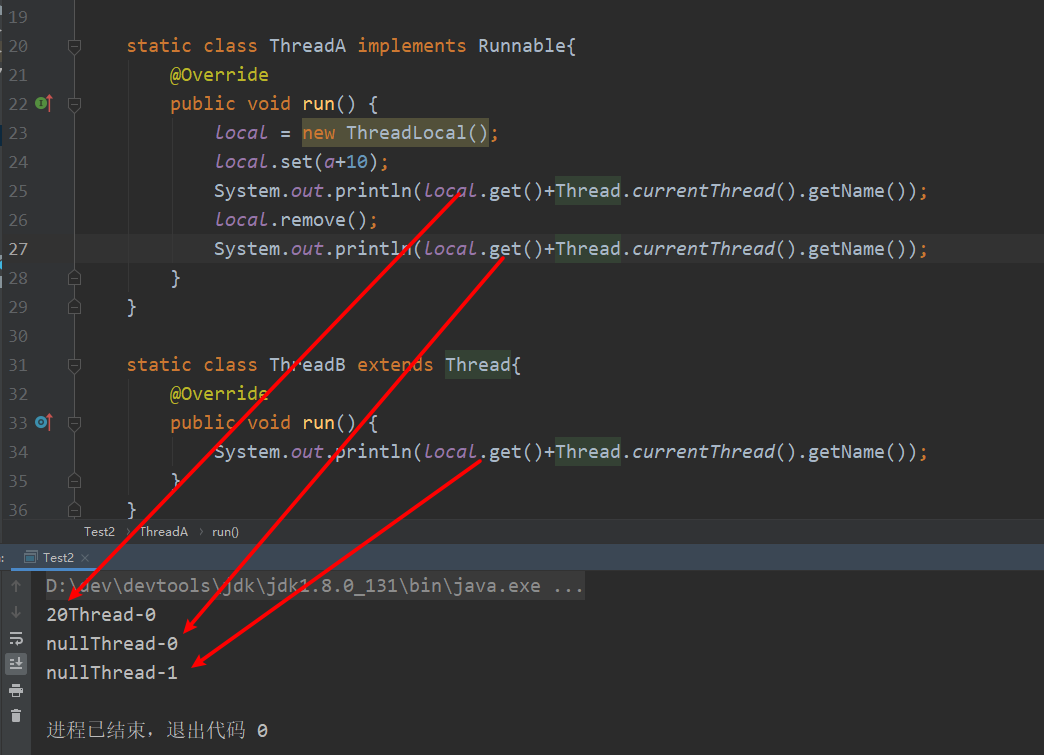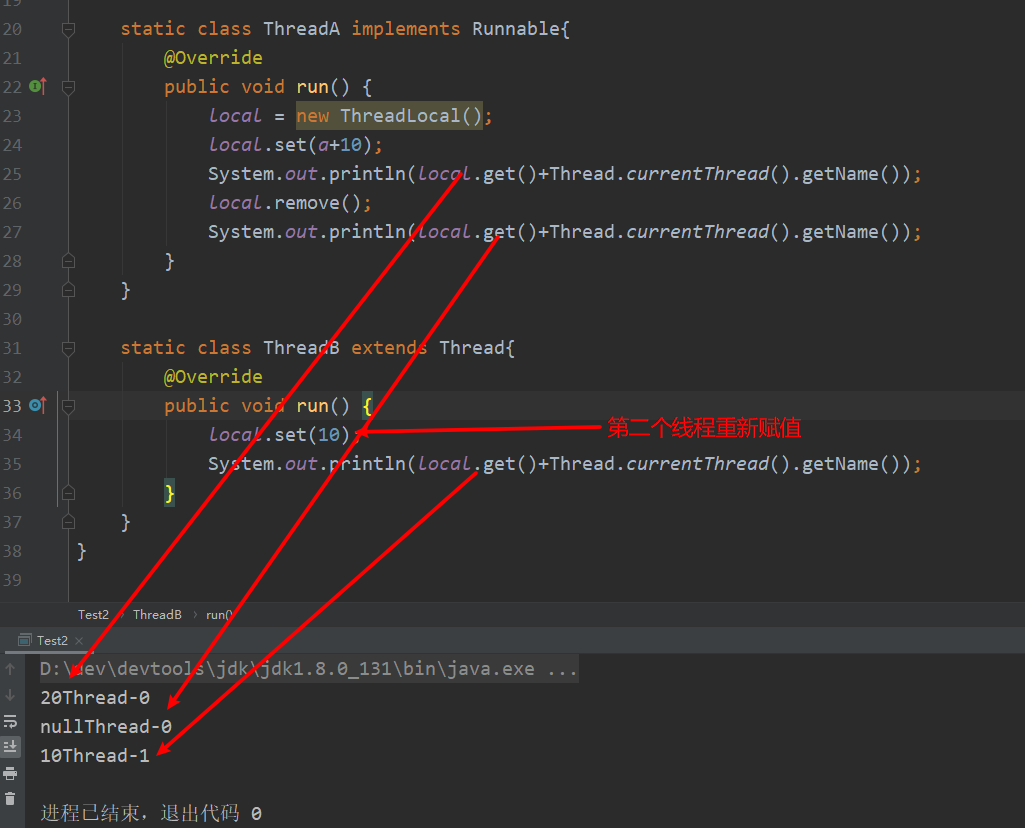local.remove();local.get()



## 源码解读get方法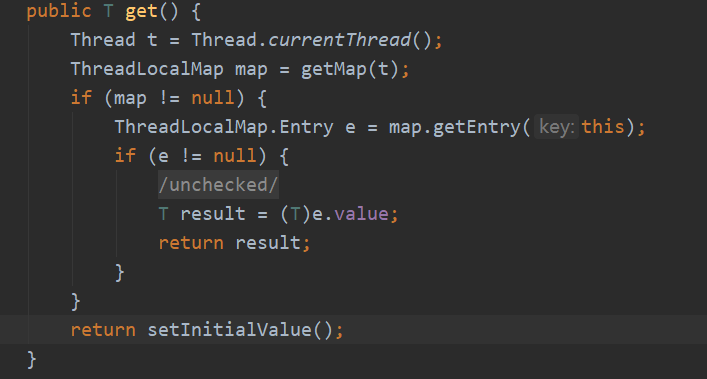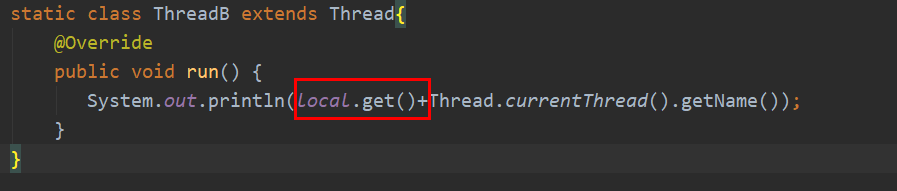Thread t = Thread.currentThread();



if (map != null) {
if (e != null) {
@SuppressWarnings("unchecked")
T result = (T)e.value;
return result;
}
}



return setInitialValue();



ThreadLocalMap.Entry e = map.getEntry(this);
if (e != null) {
@SuppressWarnings("unchecked")
T result = (T)e.value;
return result;
}



## 为什么是null？

return setInitialValue();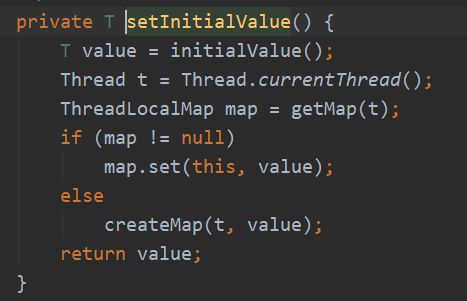T value = initialValue();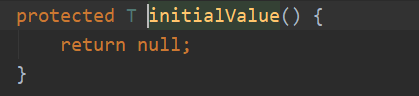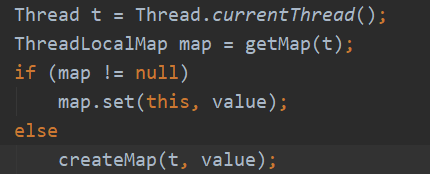createMap(t, value);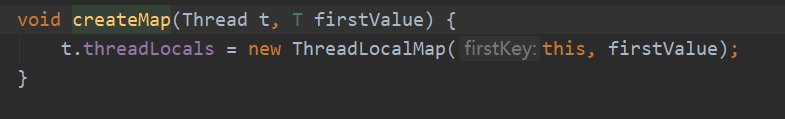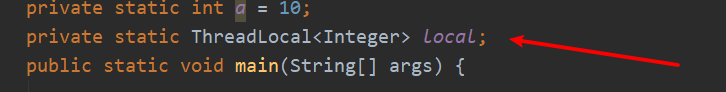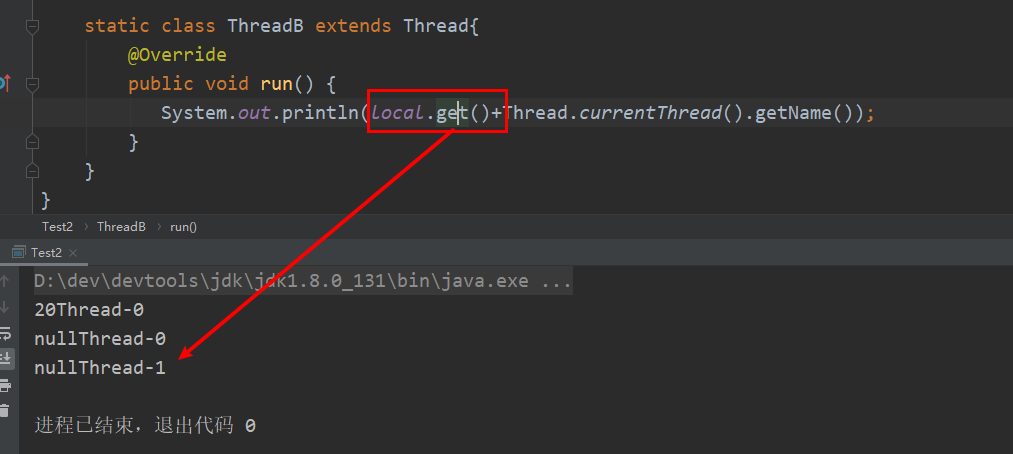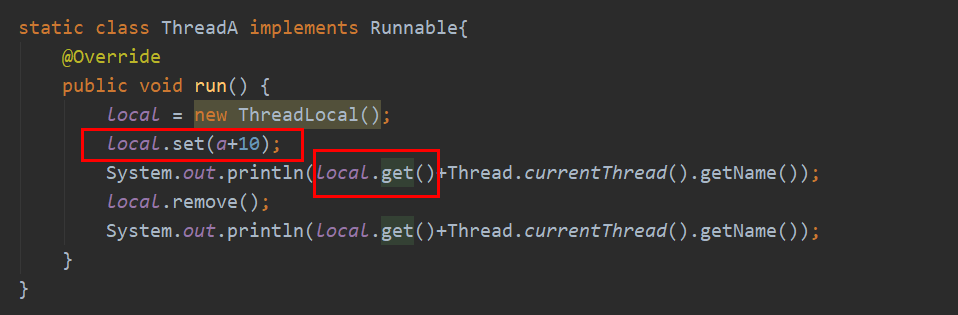## 源码解读set方法

local.set(a+10);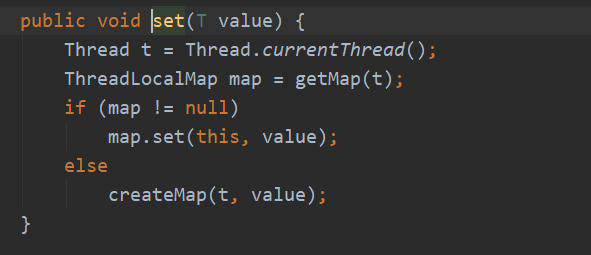createMap(t, value);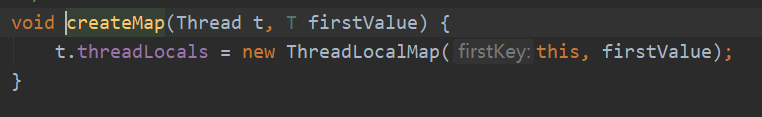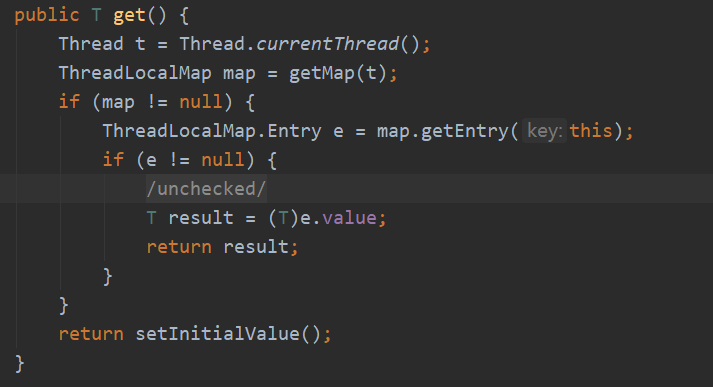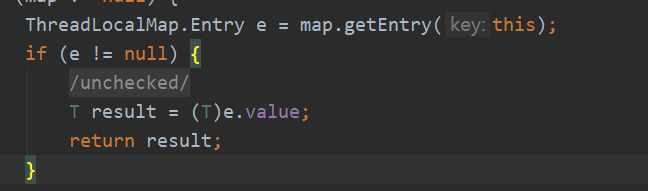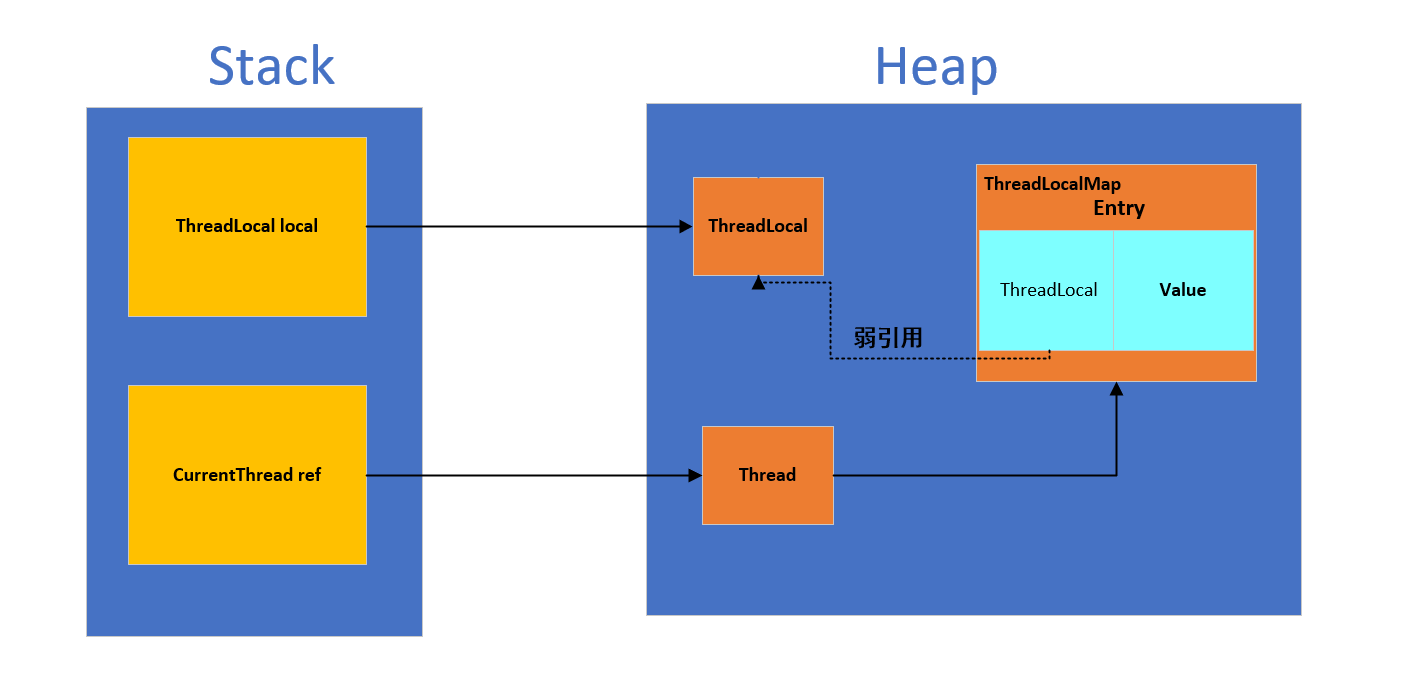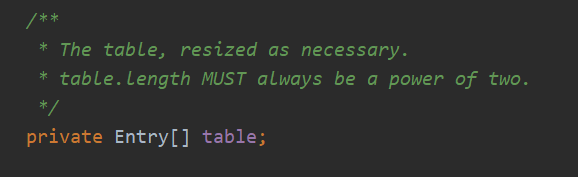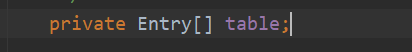### 哈希表扩容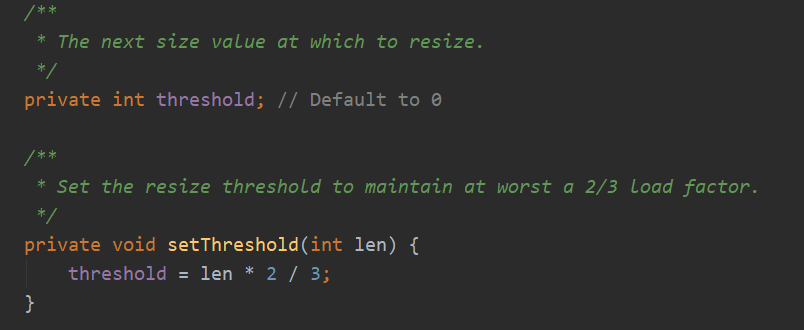The next size value at which to resize.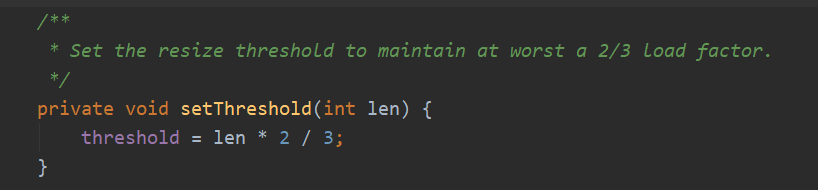Set the resize threshold to maintain at worst a 2/3 load factor.

### Entry对象是如何存储数据的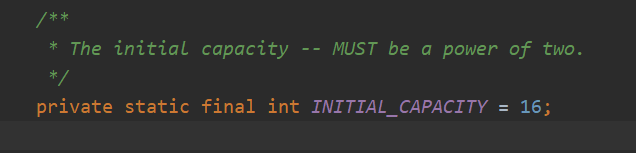private void set(ThreadLocal<?> key, Object value) {

// We don't use a fast path as with get() because it is at
// least as common to use set() to create new entries as
// it is to replace existing ones, in which case, a fast
// path would fail more often than not.

Entry[] tab = table;
int len = tab.length;
int i = key.threadLocalHashCode & (len-1);

for (Entry e = tab[i];
e != null;
e = tab[i = nextIndex(i, len)]) {

if (k == key) {
e.value = value;
return;
}

if (k == null) {
replaceStaleEntry(key, value, i);
return;
}
}

tab[i] = new Entry(key, value);
int sz = ++size;
if (!cleanSomeSlots(i, sz) && sz >= threshold)
rehash();
}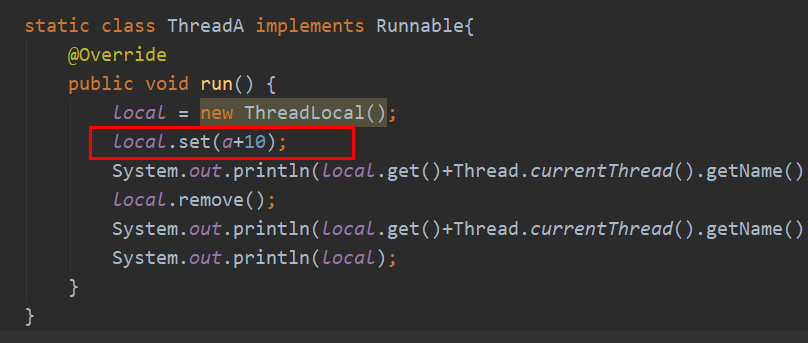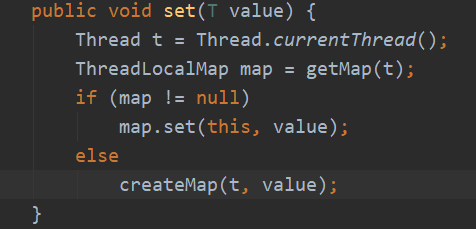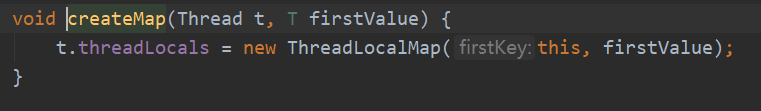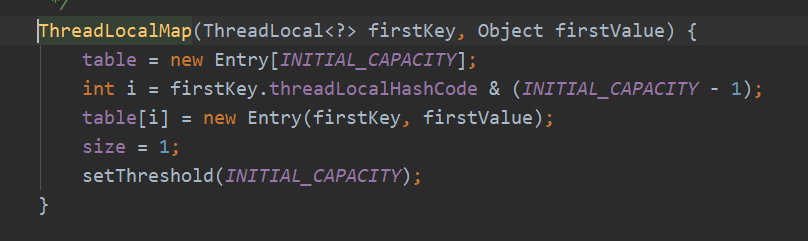table = new Entry[INITIAL_CAPACITY];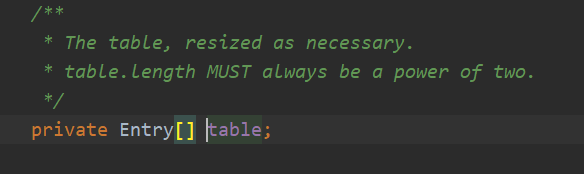int i = firstKey.threadLocalHashCode & (INITIAL_CAPACITY - 1);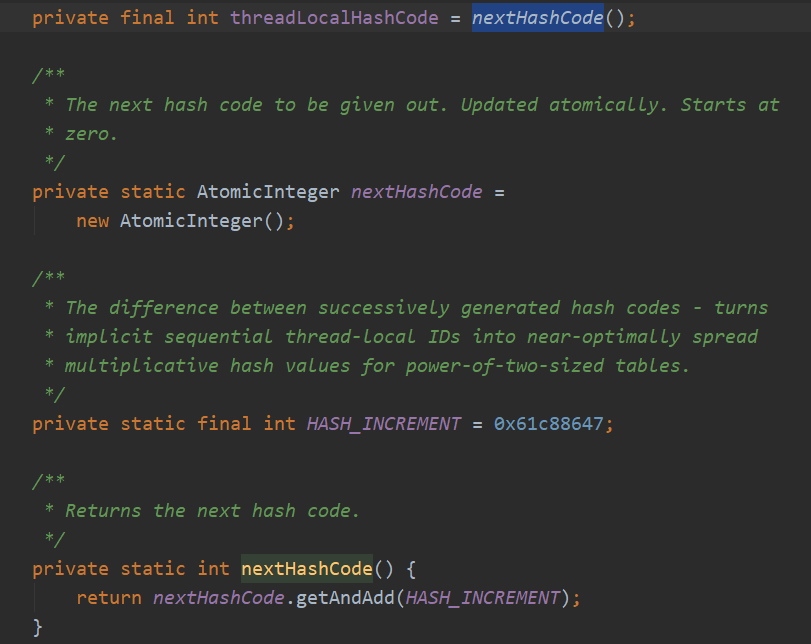firstKey.threadLocalHashCode & (INITIAL_CAPACITY - 1);



table[i] = new Entry(firstKey, firstValue);
size = 1;
setThreshold(INITIAL_CAPACITY);



table[i] = new Entry(firstKey, firstValue);



table[i]也就是Entry数组中的一个确切的位置，是要放入一个Entry对象的，这里就new了一个新的Entry对象，并把key和value传入了进去，我们看看这个Entry的构造方法以及这个Entry类的实现。

#### Entry长啥样？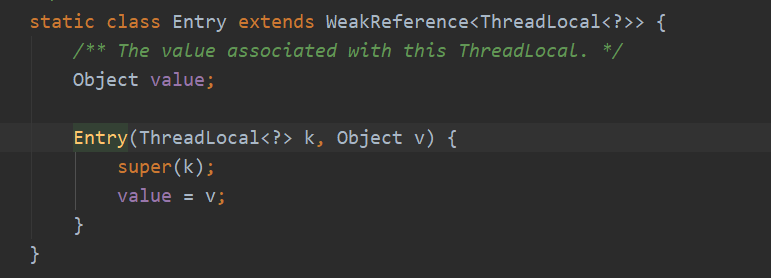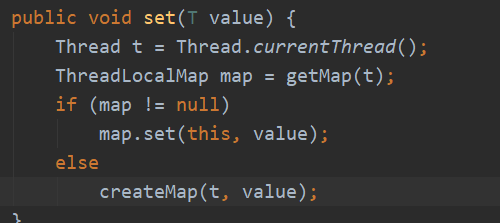private void set(ThreadLocal<?> key, Object value) {

// We don't use a fast path as with get() because it is at
// least as common to use set() to create new entries as
// it is to replace existing ones, in which case, a fast
// path would fail more often than not.

Entry[] tab = table;
int len = tab.length;
int i = key.threadLocalHashCode & (len-1);

for (Entry e = tab[i];
e != null;
e = tab[i = nextIndex(i, len)]) {

if (k == key) {
e.value = value;
return;
}

if (k == null) {
replaceStaleEntry(key, value, i);
return;
}
}

tab[i] = new Entry(key, value);
int sz = ++size;
if (!cleanSomeSlots(i, sz) && sz >= threshold)
rehash();
}



Entry[] tab = table;
int len = tab.length;
int i = key.threadLocalHashCode & (len-1);



for (Entry e = tab[i];
e != null;
e = tab[i = nextIndex(i, len)]) {

if (k == key) {
e.value = value;
return;
}

if (k == null) {
replaceStaleEntry(key, value, i);
return;
}
}



if (k == key) {
e.value = value;
return;
}



if (k == null) {
replaceStaleEntry(key, value, i);
return;
}



e = tab[i = nextIndex(i, len)])



 tab[i] = new Entry(key, value);
int sz = ++size;
if (!cleanSomeSlots(i, sz) && sz >= threshold)
rehash();



#### rehash函数是如何实现重新扩充并重新计算位置的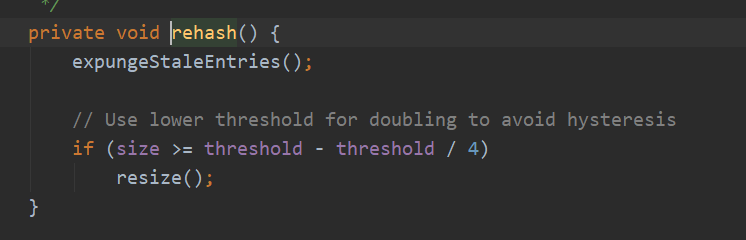### 如何获取Entry对象中的数据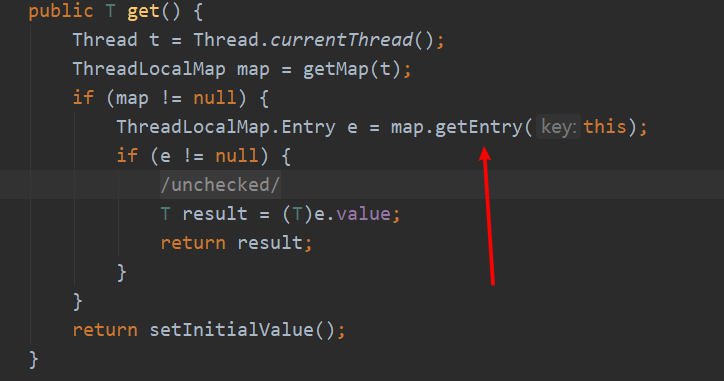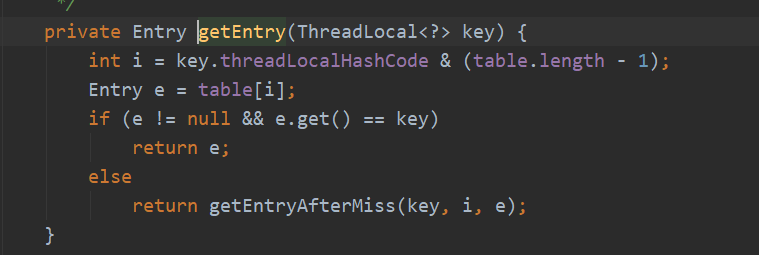## 什么是内存泄漏和内存溢出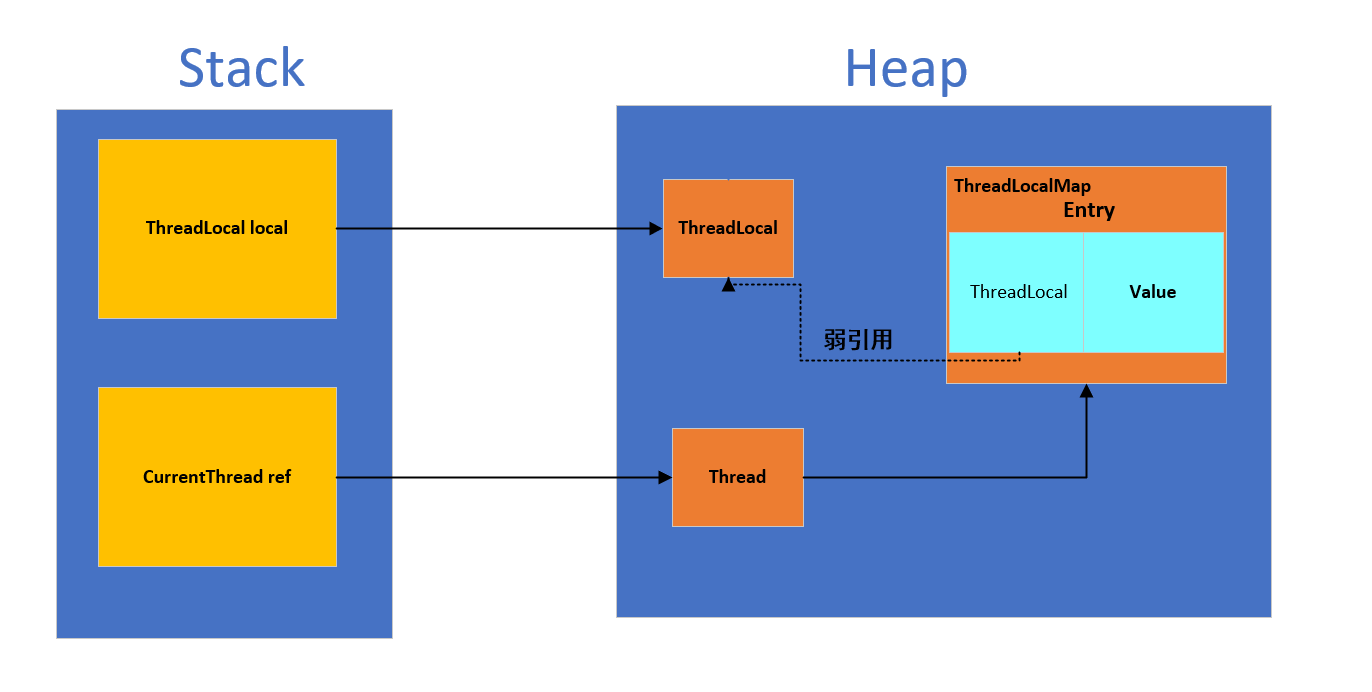## 为什么会出现内存泄漏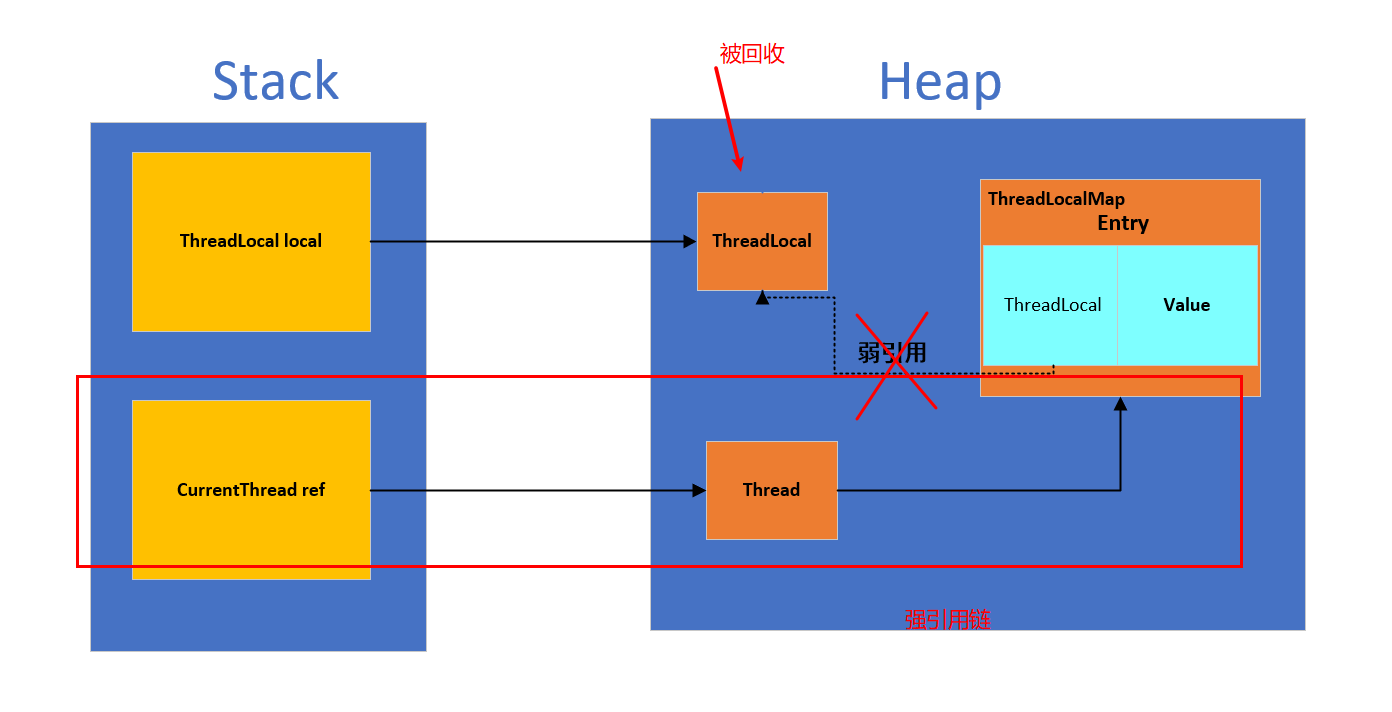## 如何解决内存泄漏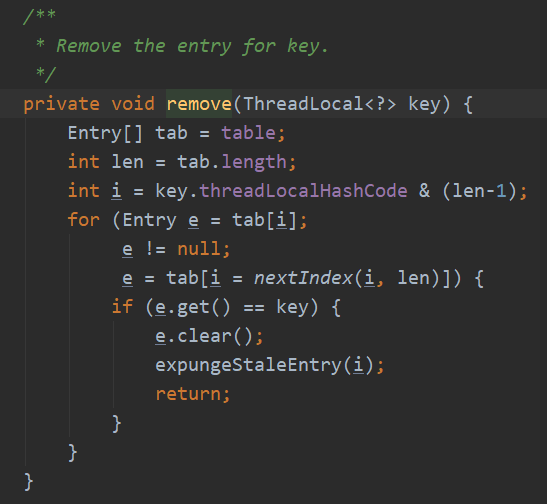# 5、总结

## 感谢阅读©️2019 CSDN 皮肤主题: 数字20 设计师: CSDN官方博客